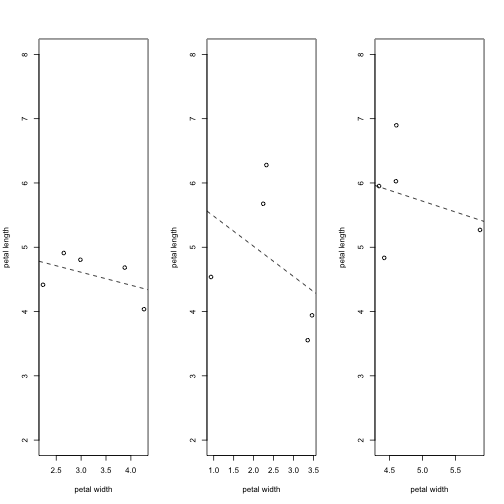# Using the by function in R

Recently, I have discovered the by function in R. With “by” you can apply any function to a data frame split by a factor. Yes, this sounds difficult, but I will show you how powerful this function is with an example.

Let’s say we have measured petal width and length of 10 individual flowers for 3 different plant species. The data frame has 3 columns (species, petal width and petal length) and 30 rows (3 species x 10 individuals). Here is the code to make this data frame:

``````# Create a data frame with petal length and petal width for 3 species
dat <- data.frame(species=c(rep(c(1,2,3), each=5)), petal.length=c(rnorm(5, 4.5, 1), rnorm(5, 4.5, 1), rnorm(5, 5.5, 1)), petal.width=c(rnorm(5, 2.5, 1), rnorm(5, 2.5, 1), rnorm(5, 4, 1)))

dat\$species <- factor(dat\$species) # make species a factor

dat
``````
```##    species petal.length petal.width
## 1        1     4.036443   4.2636530
## 2        1     4.805463   2.9856014
## 3        1     4.416011   2.2342611
## 4        1     4.910363   2.6516114
## 5        1     4.683678   3.8766098
## 6        2     6.278742   2.3196057
## 7        2     4.537683   0.9323249
## 8        2     5.676220   2.2392741
## 9        2     3.941464   3.4618104
## 10       2     3.554382   3.3538955
## 11       3     4.834811   4.4187967
## 12       3     5.952030   4.3399565
## 13       3     6.026856   4.5964251
## 14       3     5.269738   5.8714180
## 15       3     6.897427   4.6028704
```

We can use the by function to calculate the mean petal length for each of the three species.

The first argument is which data frame you want to use. Next you specify by which factor you want split your data frame. And finally you say which function you want to use. In our case this is the mean.

``````by(dat, dat\$species, function(x){
# caculate the mean petal length for each species
mean.pl <- mean(x\$petal.length)
})
``````
```## dat\$species: 1
##  4.570392
## --------------------------------------------------------
## dat\$species: 2
##  4.797698
## --------------------------------------------------------
## dat\$species: 3
##  5.796172
```

There are easier ways to calculate the mean of 3 species. But if you have understood the principle of the function, you can use it to do more complicated calculations. Here is another example, to draw a scatter plot for the petal width and petal length and drawing the regression line for each of the three species.

``````par(mfrow=c(1,3))
by(dat, dat\$species, function(x){
# caculate the mean petal length for each species
mean.pl <- mean(x\$petal.length)

# draw a plot for each speceis
plot(x\$petal.width, x\$petal.length, xlab="petal width", ylab="petal length", ylim=c(2,8))
abline(lm(petal.length ~ petal.width, x), lty=2)
})
``````You might have noticed that the output looks a bit strange. This is because the output of the by function is stored in a list. List’s are complicated but also extremely powerful. For example, a data frame needs to have the same number of entries in each row or column. A list can have entries that different in length, which can be very useful at times.

But here is a little trick to get your output back into a data frame using sapply.

``````sp.means <- by(dat, dat\$species, function(x){
# caculate the mean petal length and petal width for each species
means <- colMeans(x[,2:3])
})

# use sapply to put the data back to a matrix
sp.means2 <- t(sapply(sp.means, I))
# make a data frame
new.df <- as.data.frame(sp.means2)
new.df
``````
```##   petal.length petal.width
## 1     4.570392    3.202347
## 2     4.797698    2.461382
## 3     5.796172    4.765893
```

# Markdown – cheat sheet

I am creating my first markdown for the next blog entry. It’s very useful, because you can combine texte and R script using R. And while I was googling how to start I found this very useful cheatsheet by R markdown R Studio.